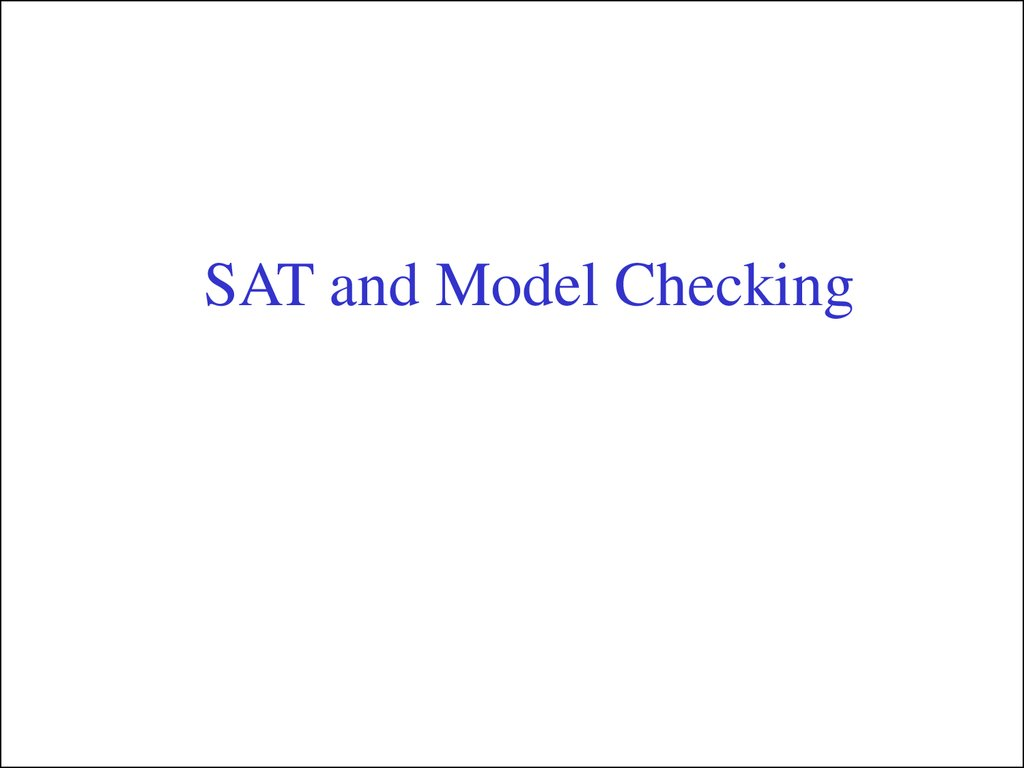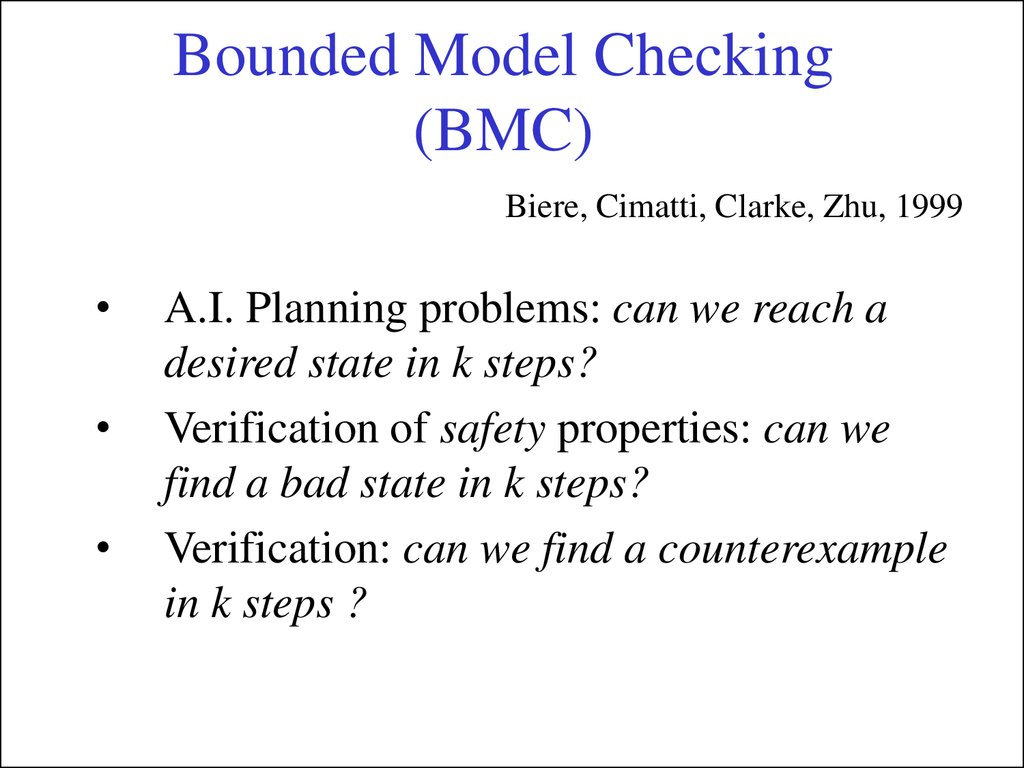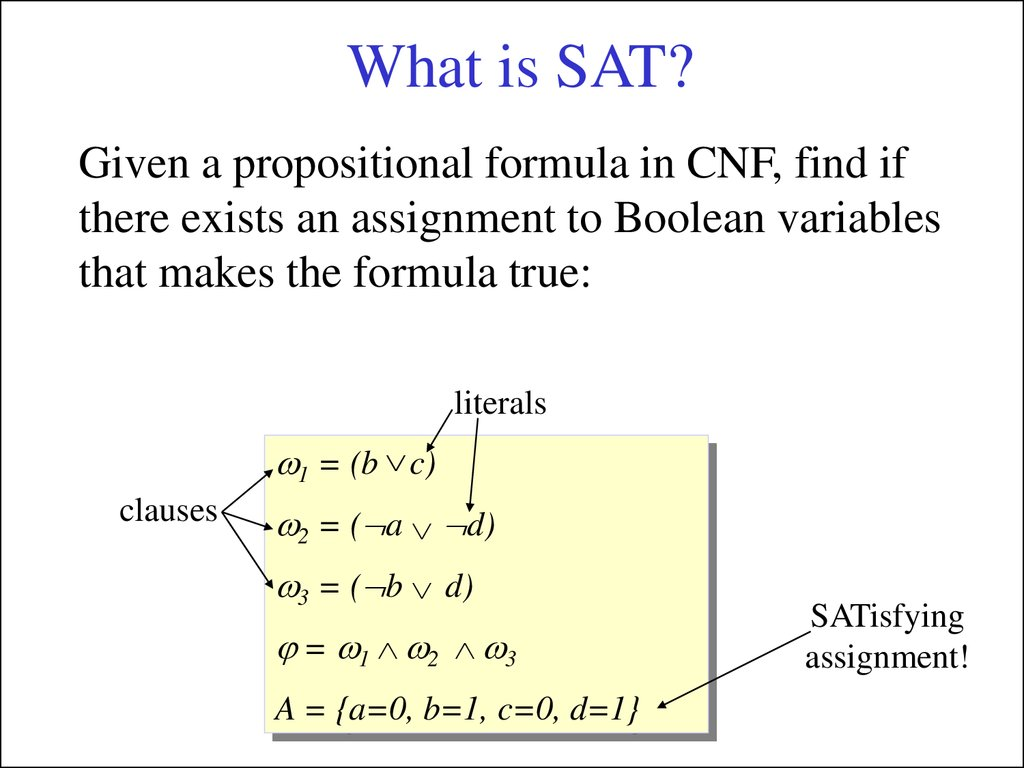# SAT and model checking

## 2. Bounded Model Checking (BMC)

Biere, Cimatti, Clarke, Zhu, 1999
A.I. Planning problems: can we reach a
desired state in k steps?
Verification of safety properties: can we
find a bad state in k steps?
Verification: can we find a counterexample
in k steps ?

## 3. What is SAT?

Given a propositional formula in CNF, find if
there exists an assignment to Boolean variables
that makes the formula true:
literals
1 = (b c)
clauses
2 = ( a d)
3 = ( b d)
= 1 2 3
A = {a=0, b=1, c=0, d=1}
SATisfying
assignment!

## 4. BMC idea

Given: transition system M, temporal logic formula f, and
user-supplied time bound k
Construct propositional formula W(k) that is satisfiable iff f is valid
along a path of length k
k 1
Path of length k:
I ( s0 ) R( si , si 1 )
i 0
Say f = EF p and k = 2, then
W(2) I ( s0 ) R( s0 , s1 ) R( s1 , s2 ) ( p0 p1 p2 )
What if f = AG p ?

## 5. BMC idea (cont’d)

AG p means p must hold in every state along any path of length k
We take
k 1
k
i 0
i 0
W(k ) ( I ( s0 ) R( si , si 1 )) pi
So
k 1
k
i 0
i 0
W(k ) I ( s0 ) R( si , si 1 ) pi
That means we look for counterexamples

## 6. Safety-checking as BMC

p is preserved up to k-th transition iff W(k) is unsatisfiable:
k 1
k
i 0
i 0
W(k ) I ( s0 ) R( si , si 1 ) p
p
p
p
s0
s1
s2
...
p
sk-1
p
sk
If satisfiable, satisfying assignment gives counterexample to the
safety property.

## 7. Example: a two bit counter

Initial state: I : l r
00
11
l ' (l r )
Transition: R :
r ' r
01
10
Safety property: AG ( l r )
(l 0 r0 )
l1 (l 0 r0 ) r1 r0
(l1 r1 )
W(2) : ( l 0 r0 )
l 2 (l1 r1 ) r2 r1 (l r )
2 2
W(2) is unsatisfiable. W(3) is satisfiable.

## 8. Example: another counter

00
11
01
10
l' l
l ' (l r )
I : l r
R:
r' r
r ' r
l
r
Liveness property: AF (l r )
Check: EG ( l r )
W(2) I ( s0 ) R( si , si 1 ) ( li ri ) loop
where
1
2
i 0
i 0
loop R( s2 , s3 ) ( s3 s0 s3 s1 s3 s2 )
W(2) is satisfiable
Satisfying assignment gives counterexample to the liveness property

## 9. What BMC with SAT Can Do

• All LTL
• ACTL and ECTL
• In principle, all CTL and even mu-calculus
– efficient universal quantifier elimination or
fixpoint computation is an active area of
research

## 10. How big should k be?

• For every model M and LTL property
there exists k s.t.
• The minimal such k is the Completeness
Threshold (CT)

## 11. How big should k be?

• Diameter d = longest shortest path from an
initial state to any other reachable state.
• Recurrence Diameter rd = longest loop-free
path.
• rd ¸ d
d=2
rd = 3

## 12. How big should k be?

• Theorem: for Gp properties CT = d
p
s0
Arbitrary path

## 13. How big should k be?

• Theorem: for Fp properties CT= rd
p
p
p
p
p
s0
Open Problem: The value of CT for general
Linear Temporal Logic properties is unknown

## 14. A basic SAT solver

Given in CNF: (x,y,z),(-x,y),(-y,z),(-x,-y,-z)
x
x
(y,z),(-y,z)
(y),(-y,z),(-y,-z)
y
-y
(z),(-z)
z
-z
()
X
()
X
z
-z
()
X
Decide()
()
(y),(-y)
-y
y
()
X
Deduce()
()
X
Resolve_Conflict()

## 15.

Basic Algorithm
Choose the next
variable and value.
Return False if all
variables are assigned
While (true)
{
if (!Decide()) return (SAT);
while (!Deduce())
}
if (!Resolve_Conflict()) return (UNSAT);
Apply unit clause rule.
Return False if reached
a conflict
Backtrack until
no conflict.
Return False if impossible

## 16. DPLL-style SAT solvers

SATO,GRASP,CHAFF,BERKMIN
A=
empty
clause?
y
UNSAT
n
Obtain conflict
clause and
backtrack
Branch:
to A
y
conflict?
n
is A
total?
y
SAT

## 17. The Implication Graph

( a b) ( b c d)
a
c
b
d
Decisions
Assignment: a b c d

## 18. Resolution

a b c
a c d
b c d
When a conflict occurs, the implication graph is
used to guide the resolution of clauses, so that the
same conflict will not occur again.

## 19. Conflict clauses

( a b) ( b c d) ( b d)
resolve
a
c
( b c )
b
Conflict!
Conflict!
( a c)
d
resolve
Conflict!
Decisions
Assignment: a b c d

## 20. Conflict Clauses (cont.)

• Conflict clauses:

Are generated by resolution
Are implied by existing clauses
Are in conflict with the current assignment
Are safely added to the clause set
Many heuristics are available for determining
when to terminate the resolution process.

## 21. Generating refutations

• Refutation = a proof of the null clause
– Record a DAG containing all resolution steps
performed during conflict clause generation.
– When null clause is generated, we can extract a
proof of the null clause as a resolution DAG.
Original clauses
Derived clauses
Null clause

## 22. Unbounded Model Checking

• A variety of methods to exploit SAT and
BMC for unbounded model checking:

Completeness Threshold
k - induction
Abstraction (refutation proofs useful here)
Exact and over-approximate image
computations (refutation proofs useful here)
– Use of Craig interpolation

## 23. Conclusions: BDDs vs. SAT

• Many models that cannot be solved by BDD
symbolic model checkers, can be solved
with an optimized SAT Bounded Model
Checker.
• The reverse is true as well.
• BMC with SAT is faster at finding shallow
errors and giving short counterexamples.
• BDD-based procedures are better at proving
absence of errors.

## 24. Acknowledgements

“Exploiting SAT Solvers in Unbounded Model Checking” by
K. McMillan, tutorial presented at CAV’03
“Tuning SAT-checkers for Bounded Model Checking” and
“Heuristics for Efficient SAT solving” by O. Strichman
Slides originally prepared for 2108 by Mihaela Gheorghiu.